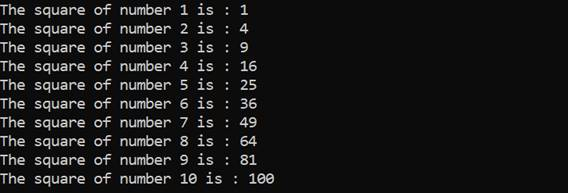# Async Streams, Static Local Functions

## Async streams

Among other features, Async streams are also one of the important features introduced in C# 8. It provides the ability to asynchronously access the stream of data that was not available earlier. Now you can create and use the streams asynchronously.

Async streams have a couple of characteristics as follows.
• It's declared with the async modifier and returns an IAsyncEnumerable<T>.
• The method contains yield return statements to return successive elements in the asynchronous stream of data.
The async and await based programming model was one of the major innovations of C# 5 and async streams is yet another enhancement on top of async and await pattern to write asynchronous code in a more intuitive way. With async streams developer now can easily implement asynchronous streams of data and also iterate asynchronously using a foreach loop. This is possible by the introduction of the new interfaces IAsyncEnumerable<T> and IAsyncDisposable<T>.

Let's have a look at the code below to understand how to work with async streams.
1. public static async Task ExecuteAsyncStream() {
2.         await foreach(var number in GenerateSequence()) {
3.             Console.WriteLine(\$ "The square of number {number.Number} is: {
4.                     number.Square
5.                 }
6.                 ");
7.             }
8.         }
9.         public static async IAsyncEnumerable < SquareNumber > GenerateSequence() {
10.             for (int i = 1; i <= 10; i++) {
12.                 yield
13.                 return new SquareNumber {
14.                     Number = i, Square = i * i
15.                 };
16.             }
17.         }
18.         public class SquareNumber {
19.             public int Number {
20.                 get;
21.                 set;
22.             }
23.             public int Square {
24.                 get;
25.                 set;
26.             }
27.         }
The output of the below code is generated as follows.IAsyncEnumerable is used with yield return and you can then consume the stream of data using an await foreach.

Although this code is extremely intuitive for the C# developer. Under the hood, the compiler generates a lot of code to implement the asynchronous behavior, but all the additional complexity is abstracted from you to let the compiler do the hard work and you can focus on writing application logic.

## Static local functions

Local functions have been introduced in C# 7 and static local function is yet another enhancement with C# 8.

Instance local function can access the variables of the parent method however static local method can't. Let's have a look at the example as follows to understand the difference between local and static local functions.
1. public static void ExecuteLocalFunction() {
2.     Console.WriteLine(InstanceLocalMethod());
3.     Console.WriteLine(StaticLocalMethod());
4. }
5. static int InstanceLocalMethod() {
6.     int x = 7;
7.     return Square();
8.     int Square() => x * x;
9. }
10. static int StaticLocalMethod() {
11.     int x = 7;
12.     return Square(x);
13.     static int Square(int num) => num * num;
14. }
Both of the methods in the above code return the same result however as you can see Method StaticLocalMethod doesn't use the instance variable x and instead had to declare local variable num. If it tries to use variable x, a compile-time error is thrown as "A static local function cannot contain a reference to x".

On the other side, regular local method InstanceLocalMethod is able to use the instance variable x.

## Summary

In this article, we have learned about Async Stream and Static local functions and why are they required. We have gone through to understand how Async Stream can be used to add power of async in the collections. StaticLocalMethod is also a new addition under local function category. Clearly these new features are the great value additions for developers.